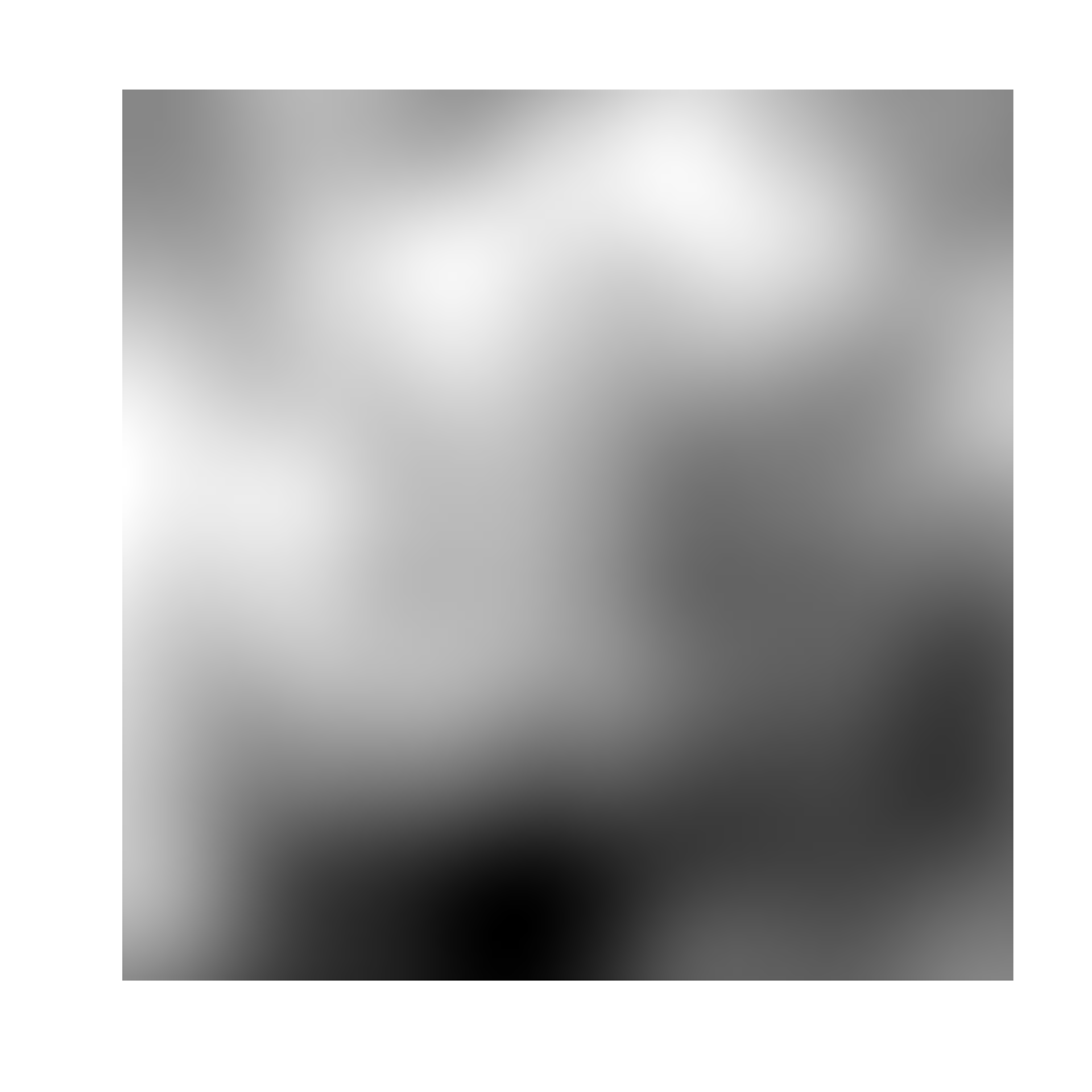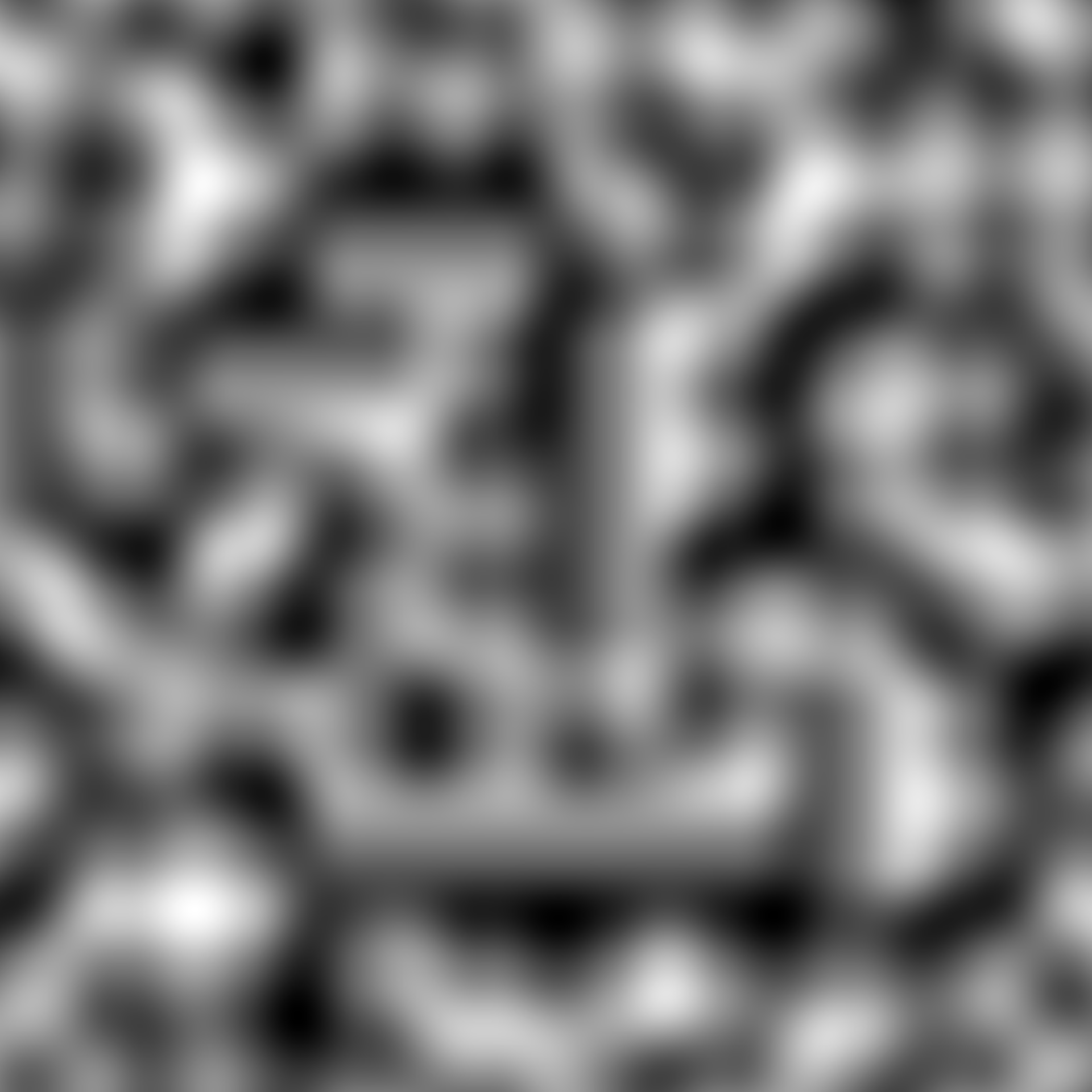This function generates either 2 or 3 dimensional perlin noise, with optional pertubation and fractality. Perlin noise is one of the most well known gradient noise algorithms and have been used extensively as the basis for generating landscapes and textures, as well as within generative art. The algorithm was developed by Ken Perlin in 1983.

noise_perlin(
dim,
frequency = 0.01,
interpolator = "quintic",
fractal = "fbm",
octaves = 3,
lacunarity = 2,
gain = 0.5,
pertubation = "none",
pertubation_amplitude = 1
)

gen_perlin(
x,
y = NULL,
z = NULL,
frequency = 1,
seed = NULL,
interpolator = "quintic",
...
)

## Arguments

dim

The dimensions (height, width, (and depth)) of the noise to be generated. The length determines the dimensionality of the noise.

frequency

Determines the granularity of the features in the noise.

interpolator

How should values between sampled points be calculated? Either 'linear', 'hermite', or 'quintic' (default), ranging from lowest to highest quality.

fractal

The fractal type to use. Either 'none', 'fbm' (default), 'billow', or 'rigid-multi'. It is suggested that you experiment with the different types to get a feel for how they behaves.

octaves

The number of noise layers used to create the fractal noise. Ignored if fractal = 'none'. Defaults to 3.

lacunarity

The frequency multiplier between successive noise layers when building fractal noise. Ignored if fractal = 'none'. Defaults to 2.

gain

The relative strength between successive noise layers when building fractal noise. Ignored if fractal = 'none'. Defaults to 0.5.

pertubation

The pertubation to use. Either 'none' (default), 'normal', or 'fractal'. Defines the displacement (warping) of the noise, with 'normal' giving a smooth warping and 'fractal' giving a more eratic warping.

pertubation_amplitude

The maximal pertubation distance from the origin. Ignored if pertubation = 'none'. Defaults to 1.

x, y, z

Coordinates to get noise value from

seed

The seed to use for the noise. If NULL a random seed will be used

...

ignored

## Value

For noise_perlin() a matrix if length(dim) == 2 or an array if length(dim) == 3. For gen_perlin() a numeric vector matching the length of the input.

## Examples

# Basic use
noise <- noise_perlin(c(100, 100))

plot(as.raster(normalise(noise)))# Using the generator
grid <- long_grid(seq(1, 10, length.out = 1000), seq(1, 10, length.out = 1000))
grid$noise <- gen_perlin(grid$x, grid\$y)
plot(grid, noise)